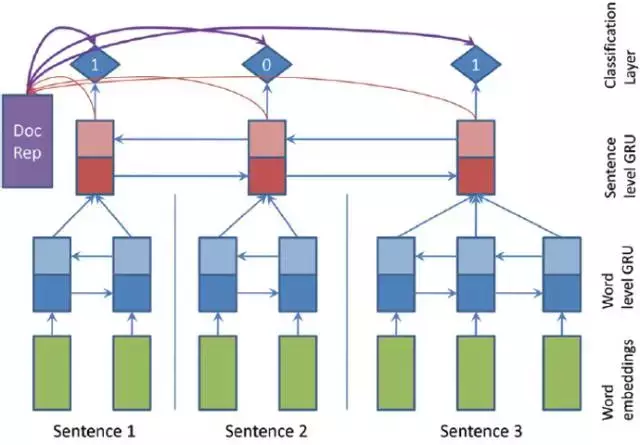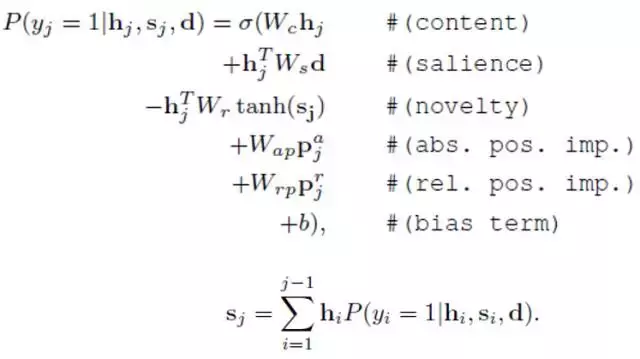Auto ByteScience AI# 用PyTorch搭建抽取式摘要系统

PyTorch是一种非常简单，优雅的动态图框架。其接口和模块的设计比较清晰，能够帮助你快速实现自己的想法。下面借用李飞飞教授公开课cs231n第8讲Deep Learning Software的内容介绍下动态图相比静态图的一些优势。当然静态图也有自己的优势，这里不加讨论，大家可以详细看下这一讲的内容帮助你挑选心仪的框架。下面介绍静态图和动态图的一个重要区别：循环和条件判断。

① 条件判断

``````if z > 0:
...else:
...``````

Tensorflow版本:

`tf.cond(tf.less(z,0),f1,f2)`

② 循环

PyTorch版本

`for t in range(T):    ...`

Tensorflow版本:

`tf.fold1(f,arg1,arg2)`① 模型训练

`net = SummaRuNNer(config)net.cuda()`

``````# Loss and Optimizercriterion = nn.BCELoss()

` outputs = net(sents)`

`optimizer.zero_grad()loss = criterion(outputs, labels)loss.backward()`

``````# gradient clipping     torch.nn.utils.clip_grad_norm(net.parameters(), 1e-4)
optimizer.step()``````

`torch.save(net.state_dict(), args.model_file)`

② 模型测试

``````net = SummaRuNNer(config).cuda()
doc = docs
x, y = prepare_data(doc, word2id)
sents = Variable(torch.from_numpy(x)).cuda()
outputs = net(sents)``````

③ 网络搭建

PyTorch搭建网络一般需要继承nn.Module这个类，并实现里面的forward()函数。虽然一开始感觉不太灵活，但nn.Module为我们封装了一些操作，为我们编程带来便利，例如net.parameters()可得到网络所有需要训练的参数。另外，这种方式也让代码可读性更高。下面是利用  nn.Module搭建网络的代码框架：

``````class SummaRuNNer(nn.Module):
def __init__(self, config):
super(SummaRuNNer, self).__init__()
...        def forward():
...``````

``````# word level GRUword_features = self.word_embedding(x)
word_outputs, _ = self.word_GRU(word_features)``````

``````# sentence level GRU# 句子级别RNN的输入是上一层词级别RNN的隐层做average poolingsent_features = self._avg_pooling(word_outputs, sequence_length)
sent_outputs, _ = self.sent_GRU(sent_features.view(1, -1, self.sent_input_size))``````

``````# document representationdoc_features = self._avg_pooling(sent_outputs, [[x.size(0)]])
doc = torch.transpose(self.tanh(self.fc1(doc_features)), 0, 1)``````

``````# classifier layeroutputs = []
sent_outputs = sent_outputs.view(-1, 2 * self.sent_GRU_hidden_units)# 初始化当前摘要表示        s = Variable(torch.zeros(100, 1)).cuda()# 分类层for position, sent_hidden in enumerate(sent_outputs):
h = torch.transpose(self.tanh(self.fc2(sent_hidden.view(1, -1))), 0, 1)
position_index = Variable(torch.LongTensor([[position]])).cuda()
p = self.position_embedding(position_index).view(-1, 1)

content = torch.mm(self.Wc, h)
salience = torch.mm(torch.mm(h.view(1, -1), self.Ws), doc)        # 这里用tanh(s)而不是直接用s的原因是让s的值保持在一定体量
novelty = -1 * torch.mm(torch.mm(h.view(1, -1), self.Wr), self.tanh(s))
position = torch.mm(self.Wp, p)
bias = self.b
Prob = self.sigmoid(content + salience + novelty + position + bias)
s = s + torch.mm(h, Prob)

 Chen K Y, Liu S H, Chen B, et al. Extractive broadcast news summarization leveraging recurrent neural network language modeling techniques[J]. IEEE/ACM Transactions on Audio, Speech and Language Processing (TASLP), 2015, 23(8): 1322-1334.  Mihalcea R, Tarau P. TextRank: Bringing Order into Text[C]//EMNLP. 2004, 4: 404-411.     Cheng J, Lapata M. Neural summarization by extracting sentences and words[J]. arXiv preprint arXiv:1603.07252, 2016.  Nallapati R, Zhai F, Zhou B. SummaRuNNer: A recurrent neural network based sequence model for extractive summarization of documents[J]. hiP (yi= 1| hi, si, d), 2017, 1: 1.